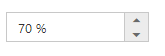# Getting Started

24 Nov 20173 minutes to read

## Control Initialization

• Open the command prompt in the folder ember-app or the folder in which the application is created.

• Use the command ember generate route percentage/default to create template `default.hbs` file in templates folder and router `default.js` file in routes folder. It also add the routing content in `router.js`.

• Use below code in `default.hbs` in templates folder to render the Textboxes.

• HTML
• ``{{ej-percentagetextbox id="percentage" e-value=model.percent}}``
• Use the below code in `default.js` in routes folder to bind the model to the Textboxes.
• HTML
• ``````export default Ember.Route.extend({
model(){
return {
percent : 70
}
}``````

## Running the application

• To run the application, execute below command.
• JAVASCRIPT
• ``ember serve``
• Browse to http://localhost:4200 to see the application. And navigate to PercentageTextbox sample (http://localhost:4200/percentage/default). The component is rendered as like the below screenshot. You can make changes in the code found under app folder and the browser should auto-refresh itself while you save files.## Decimal Places

The decimalPlaces property specifies number of values allowed after the decimal point. The default value of decimalPlaces property is 0. i.e., By default you cannot specify decimal value in PercentageTextbox. We need to add this property to allow decimal values.

• HTML
• ``{{ej-percentagetextbox id="PercentageTextbox" e-value=model.percent e-decimalPlaces = model.decimals}}``

## Strict Mode Support

PercentageTextbox allows you to use the strict mode option by setting the enableStrictMode property. You can set the minValue and maxValue to the controls to enable strict mode functionality. Default value of this property is false. When the textbox value exceeds the maxValue, it restricts the exceeded value and returns the maxValue. Likewise when the textbox value goes below minValue, it restricts the new value and returns the minValue. When this property is true, it will not restrict the specified value and an error class will be added to indicate wrong value is provided to the PercentageTextbox.

### Configure Strict Mode Support

The following steps explain the implementation of enableStrictMode in PercentageTextbox .

• HTML
• ``{{ej-percentagetextbox id="percent"  e-value=model.percent e-enableStrictMode = true e-minValue = model.min e-maxValue = model.max e-decimalPlaces = model.decimals}}``
• HTML
• ``````export default Ember.Route.extend({
model(){
return {
percent : 35,
min: 10,
max : 30
}
}
});``````

## Increment Step

The incrementStep property is used to increase or decrease the amount of value in the PercentageTextbox control.

### Configure Increment Step

The following steps explain the implementation of incrementStep in PercentageTextbox.

• HTML
• ``{{ej-percentagetextbox id="percentage" e-incrementStep = model.step e-value=model.percent e-enableStrictMode = true e-minValue = model.min e-maxValue = model.max e-decimalPlaces = model.decimals}}``
• HTML
• ``````export default Ember.Route.extend({
model(){
return {
percent : 35,
min: 10,
max : 30,
step: 5
}
}
});``````